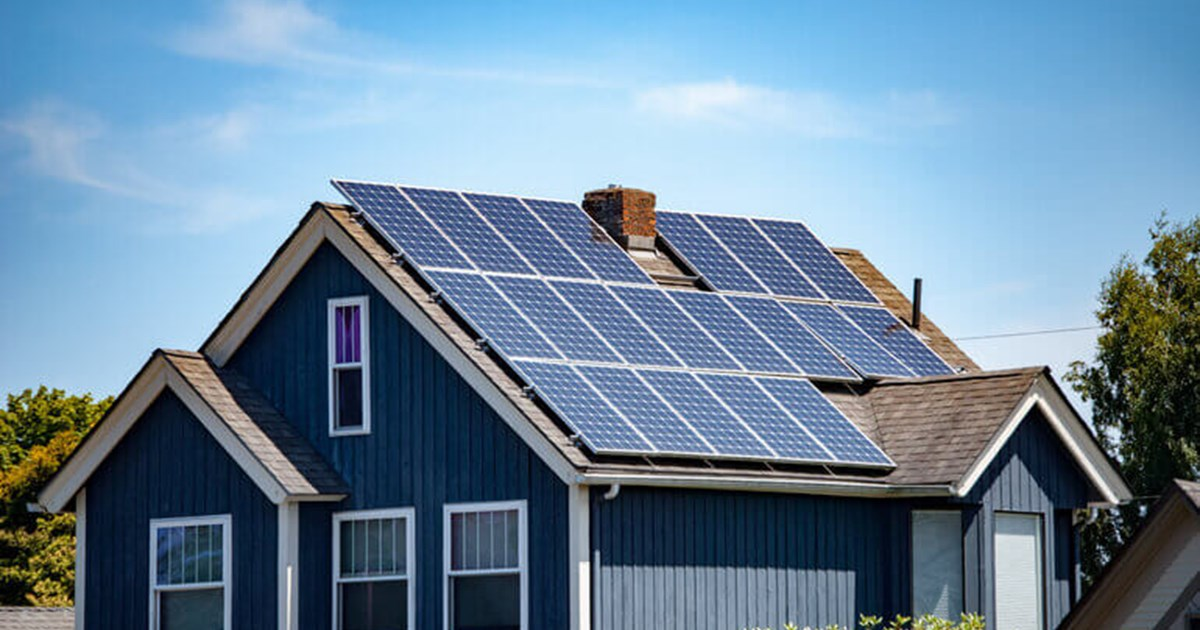## Introduction

In the last few decades, solar panels have quickly become very popular. This is partly because more people care about the environment and want to save money on energy bills by switching to cleaner, more natural ways to heat, cool, and make electricity. If you’re thinking about making the switch, you might be wondering how many solar panels it takes to power a house.

Even though the answer is complicated, if you talk to a professional, they will probably also take care of this step. Before you can figure out how many solar panels you need, you will need to know how much energy your home uses, what more space you have on your roof for solar panels, and how much sun your home gets each year. How much power will your photovoltaic (PV) solar panels make, and how well will they work overall.

### How Do I Decide The Proper Number Of Solar Panels For My Home?

Energy Sage says that to figure out how many solar panels are needed to power a house, you need a formula that considers the annual energy use, the wattage of the panels, and the production ratios.

### How Much Energy Is Used Each Year

The first step is to determine how much electricity your whole house uses in a year. This is called your annual electricity consumption. This number, given in kWh, includes all the places in your home where electricity is used, such as small and large appliances, air conditioners, lights, air purifiers, and water heaters. The US Energy Information Administration says the average home uses about 11,000 kWh of electricity each year (EIA).

### Solar Panel Wattage

Solar panels may look the same, but they aren’t. You’ll need to know how many watts the panels you want to install have. Wattage is a way to measure how much electricity the panel can make. Most solar panels have a range of power from 250 to 400 watts, so the average wattage of a panel is 300.

## Ratios Of ProductionEnergy Sage says that a solar panel system’s production ratio is the estimated energy output over time (in kWh) divided by the actual size of the system (in W). You might think that what you put in is what you get back. This isn’t true, though, because the amount of sunlight that hits your home can change.

The production ratio of a 10 kW system that makes 16 kWh of electricity per year is 1.6 (16/10 = 1.6). This ratio is possible in places like Hawaii, where long days and constant sunshine are the norms, but the average production ratio in cloudy, rainy New England may only be 1.2.

## A Mathematical Formula For Determining The Number Of Solar Panels Required

Energy Sage uses this formula to figure out how many solar panels you need:

• Number of panels = size of the system, production rate, and wattage of each panel
• Using the numbers we have so far, we come up with:
• 11,000 kW / 1.6 / 300 W = panel count

For the project to be done, you’ll need between 20 and 25 solar panels. You can use the same formula to figure out how many solar panels you need to power your home, and you can also take the easy way out and look at your energy bill to figure out how much you’ll need.

### Conclusion

If you don’t want to do the Maths, look at your utility bills to see how much energy you use. This lets you multiply your energy use by the number of hours your home gets direct sunlight, then divide the result by the wattage of the solar panels you’re thinking about buying.